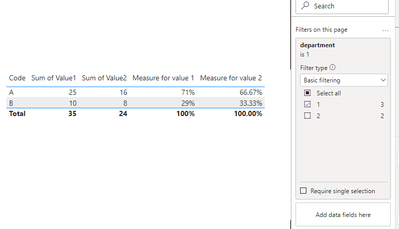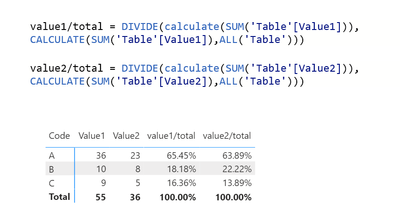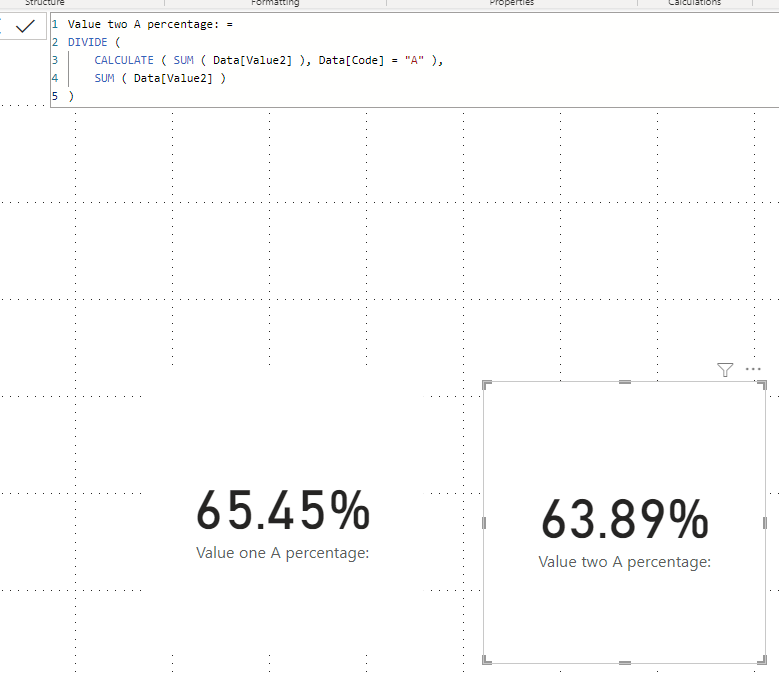cancel
Showing results for
Did you mean:Helper IV

## sumif or equivalent

I am trying to get the percentage of a value in a column based on one condition.
In excel I would use something along SUMIF or SUMIFS to get the value I want and would divide it by the total without filter.
Example

 Code Value1 Value2 A 12 9 B 10 8 A 13 7 C 9 5 A 11 7

I would like a measure that would give:
Measure for value 1: A = 65.5% (12+13+11) divided by 55
Measure for value 2: A = 63.9% (9+7+7) divided by 36

1 ACCEPTED SOLUTIONCommunity Support

Hi @Cyrilbrd ,

Here is my example:``````Measure for value 1 =
var _a = SUM('Table'[Value1])
var _b = CALCULATE(SUM('Table'[Value1]),REMOVEFILTERS('Table'[Code]))
return DIVIDE(_a,_b)

Measure for value 2 =
var _a = SUM('Table'[Value2])
var _b = CALCULATE(SUM('Table'[Value2]),REMOVEFILTERS('Table'[Code]))
return DIVIDE(_a,_b)``````

Output:Best Regards,

Jianbo Li

If this post helps, then please consider Accept it as the solution to help the other members find it more quickly.

10 REPLIES 10Helper IV

@v-jianboli-msft Thanks for the input, it works as requested.
Interrestingly I would have never thought of REMOVEFILTER.

The solution works with both slicers and filters.
Thanks.Community Support

Hi @Cyrilbrd ,

Here is my example:``````Measure for value 1 =
var _a = SUM('Table'[Value1])
var _b = CALCULATE(SUM('Table'[Value1]),REMOVEFILTERS('Table'[Code]))
return DIVIDE(_a,_b)

Measure for value 2 =
var _a = SUM('Table'[Value2])
var _b = CALCULATE(SUM('Table'[Value2]),REMOVEFILTERS('Table'[Code]))
return DIVIDE(_a,_b)``````

Output:Best Regards,

Jianbo Li

If this post helps, then please consider Accept it as the solution to help the other members find it more quickly.Helper IV

Allow me to rephrase it.

 Code Value1 Value2 A 12 9 B 10 8 A 13 7 C 9 5 A 11 7

With the code being ten of thousand or rows.
Filters at page level as to show data only for selected codes is existing.
Slicer to show locations, or statuses or department are also existing and are applied via interactions.
CUrrently both solutions are showing incorrect info.
The solution of @Padycosmos is the closest but works only if all filters and slicers are off.
Code A ratio for value 1 could be total COG (Cost Of Goods) in a specific location the it could be for all locations.
Thus allowing comparison of KPI.
If I was to "Show value as Percent of grand total", then it would give me a percenatge that would not work unless ALL codes were displayed.
Showing only selected codes would render "Show value as Percent of grand total" useless.Solution Sage

Hope this helpsHelper IV
``Measure 2 = divide( calculate(sum(Main[Value1]),Main[code_cleaned] ="88-NL-A"),sum(Main[Value1]))``

But i still get an incorrect result.Solution Sage

You need to use the formula in the screenshot exactly as shown. The formula you have typed is incorrect.Super User

Hi,

Please check the below picture and the attached pbix file.If this post helps, then please consider accepting it as the solution to help other members find it faster, and give a big thumbs up.Helper IV

@Jihwan_Kim Thanks for the proposal.
I am getting a : The True/False expression does not specify a column. Each True/False expressions used as a table filter expression must refer to exactly one column.
I used this:

``Measure 2 = divide( calculate(sum(Main[Value1]),code_cleaned ="88-NL-A"),sum(Main[Value1]))``

Did I omit somthing?Super User

Hi,

``code_cleaned``

?

If this post helps, then please consider accepting it as the solution to help other members find it faster, and give a big thumbs up.Helper IV

Context:

The data comes from a server.
The data may need "cleaning" such as to ensure that the code is corrected.
Code_cleaned is the code minus unwanted.
So far the measure returns a 100% for the code of interest and percentages in excess to 100% for all other codes.
I only noticed your proposal this morning, and it works as displayed.
The new issue is I have several slicers and Filters.
Example, location, status, department are filters that I require.
What would be the best approach to ensure that a dashboard with specifc filters would display the appropriate result?